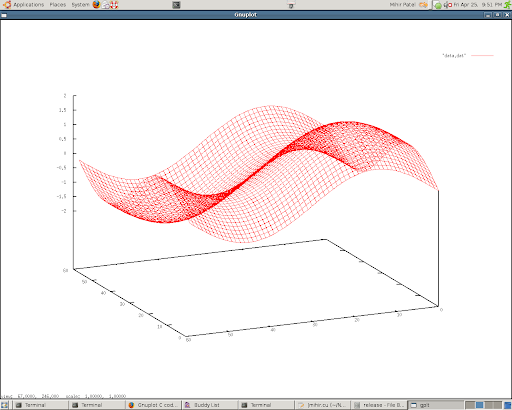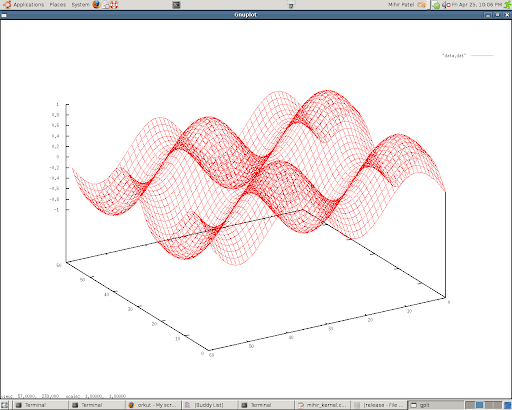## Friday, April 25, 2008

### 2D Sine wave on GPU +CUDA Programming(x_amp =1, y_amp=1, x_wave=1, y_wave=1)

Worked out 2d arrays to produce sine wave using CUDA programming on GPU.

Gnuplot is used for plotting the image.

The time saving for computation is high (haven't got a numerical value yet)..(x_amp =0.5, y_amp=0.5, x_wave=2, y_wave=2)

-- Mihir Patel.